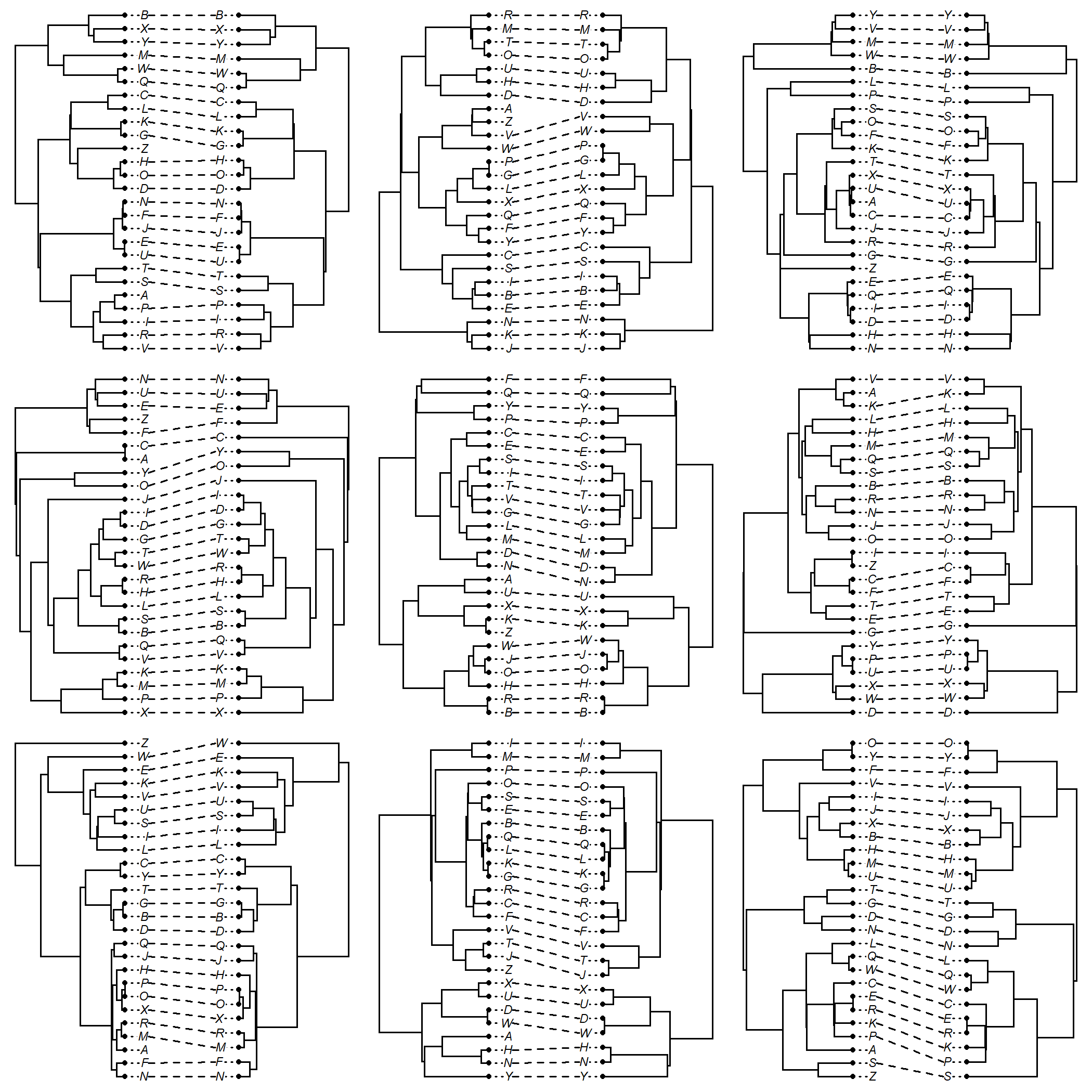## Thursday, June 18, 2020

### Pruning tips from a `"multiPhylo"` object

`drop.tip` is a popular ape function for pruning taxa from an object of class `"phylo"`.

phytools likewise has a number of different `drop.tip.______` functions (not methods, as `drop.tip` is not a registered S3 method) to prune tips from the various special object classes of the package, such as `"simmap"`, `"contMap"`, and `"densityMap"` objects.

It's fairly easy to apply `drop.tip` to a set of trees in a `"multiPhylo"` object, but the following simple wrapper function vectorizes `drop.tip` for the `"multiPhylo"` object class.

``````drop.tip.multiPhylo<-function(phy, tip, ...){
if(!inherits(phy,"multiPhylo"))
stop("phy is not an object of class \"multiPhylo\".")
else {
trees<-lapply(phy,drop.tip,tip=tip,...)
class(trees)<-"multiPhylo"
}
trees
}
``````

Let's see how it works. Just to be sure that the function is doing what it's supposed to be doing, I'm randomizing the tip order in my set of trees as well as compressing the tip labels of the `"multiPhylo"` object using `ape::.compressTipLabel`.

``````library(phytools)
trees<-replicate(9,pbtree(n=26,tip.label=sample(LETTERS)),
simplify=FALSE)
class(trees)<-"multiPhylo"
trees<-.compressTipLabel(trees)
trees
``````
``````## 9 phylogenetic trees
``````

OK, now let's try to prune the taxa `"A"` and `"Z"` from all our trees:

``````pruned<-drop.tip.multiPhylo(trees,c("A","Z"))
``````

To make sure it works, I'll plot all of the original and pruned trees using the phytools `cophylo` plotting method:

``````par(mfrow=c(3,3))
nulo<-mapply(function(x,y) plot(cophylo(x,y,rotate=FALSE),
fsize=0.7),x=trees,y=pruned)
``````That's OK.